Data science is a concept of combining statistics, data analysis, machine learning, and other mathematical skills and artificial intelligence techniques in order to extract knowledge from a set of data.

Knowing how fast the technology is progressing and how much information we create every day, we are going to need all of these techniques that the Data Science concept “employs” in order to extract the right information that will help us improve those new technologies and increase the percentage of truth in the data that we create in order to improve our day to day lifestyle that is so depended on these things.

We’ve written another blog post about the top 10 Machine Learning books you should read before you start it.

### Here are two important and useful books to buy and read:

Pattern Recognition and Machine Learning Buy from Amazon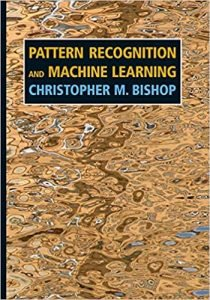Hands-On Machine Learning with Scikit-Learn and TensorFlow Buy from Amazon## Essential mathematical skills for every data scientist

As we’ve mentioned in the intro, Data Science is a concept of combining different techniques, from different mathematical and artificial intelligence fields in order to extract valuable knowledge from a certain set of data.

Data Science is a huge area of study, and it consists of many subfields. In this post we are going to show you, what are the essential mathematical skills that you need to be familiar with before you dive into learning the basic Data Science techniques and algorithms that will help you manipulate different data formats.

## Discrete Math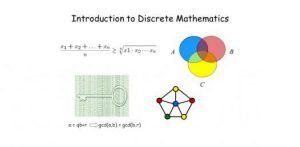Image 1: Discrete Mathematics to Become Data Scientist

All of the data nowadays is computed using computers with high processing power that allows them to make a huge number of computations in a short period of time.

The concepts studied in the field of Discrete Math are the main idea and moving power behind these computers. In Discrete Math, you will learn the basics of how data is connected and related.

### The main concepts that you will get familiar are:

• Sets, subsets, power sets
• Counting functions
• Proof techniques like induction, contradiction
• Basics of inductive, deductive, and propositional logic
• Data structures like arrays, stacks, queues, hash tables, trees
• Graphs and their properties like: connected components, degree, graph coloring, graph traversal, searching methods and algorithms
• Growth of functions and O(n)notation concept
• Intro into Combinatorics (Combination, Variation, Permutation)

You will use these concepts a lot in your day to day work. For example, the Graphs are used in any sort of network analysis, the growth of functions and O(n) notation concept is used if you want to calculate the time and space complexity of a certain algorithm that you use or create yourself. Data structures will help you decide which of those structures is the best to use in order to create an algorithm that will execute fast and will not require huge computational power, combinatorics will help you understand probability and it’s distributions fairly easy, etc.

## Calculus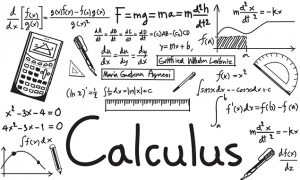Image 2: Calculus Mathematics

This field of mathematical studies is the backbone of many Data Science, Machine Learning, Deep Learning techniques that we are using in every little model we create. That means that knowledge if the main concepts in this field are required.

### The main concepts that you are going to learn here are:

• Functions of a single variable, limit, differentiability
• Functions of multiple variables, limit, continuity, partial derivatives
• L’Hospital’s rule (used in special cases when doing differentiability)
• Maxima and minima
• Product and chain rule
• Taylor’s/Maclaurin’s series, infinite series summation and integration
• M value-theorems of integral calculus
• Beta and gamma functions

You will have to have a good understanding of all of these concepts if you want to know how the basic techniques of Data Science/Machine Learning and Deep Learning work behind a single line of code that you type in order to call them and to solve your problem.

Derivatives, Integrals, Chain rules will help you understand how logistic regression is implemented or how the gradient descent method finds minimal loss function and so on.

Statistics (Fourth Edition) 4th Edition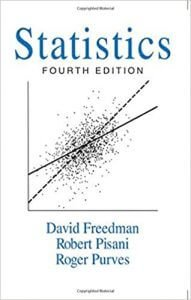## Linear Algebra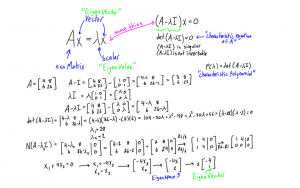Image 3: Linear Algebra

Linear algebra is basically the backbone of understanding how you should organize the data in order for the Machine Learning algorithms to use it and produce some output. Its concepts are used anywhere you can imagine.

### The main concepts you are going to learn in this field of study are:

• Matrices and vectors: scalar multiplication, linear transformation, transpose, calculating the determinant
• Inner and outer products, matrix multiplication rule
• Different matrices types like: square matrix, identity matrix, triangular matrix, an idea about sparse and dense matrix, unit vectors, symmetric matrix
• Matrix factorization concept, Gauss-Jordan elimination, linear system of equation
• Vector space, basis, span, orthogonality, orthonormality, linear least square

As we’ve you are going to use this concept everywhere. All neural network algorithms use linear algebra techniques, for example when you are doing Max pooling on an image using Convolutional Neural Networks (CNNs).

The process of Max pooling uses matrices, and it is a discretization process that objective is to down-sample an input representation (image, hidden-layer output matrix, etc.), reducing its dimensionality and allowing for assumptions to be made about features contained in the sub-regions binned.

It is a very interesting and important concept that we are going to explain in some of our future posts.

## Probability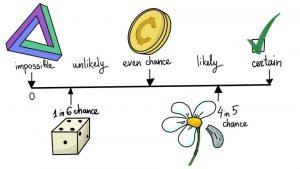Image 4: Probability to Learn Essential Mathematical Skills

Probability is a numerical description of how likely an event is to occur and happen or how likely it is that a proposition is true. Probability is a number between 0 and 1, where, roughly speaking, 0 indicates impossibility and 1 indicates certainty.

This being said, we can see that probability is in the heart of every prediction model we build, because by the end of the day, all we do is try to predict some value for some information at a certain time point. The likeliness of that information having that value is a probability.

### The main concepts that you are going to use in probability are:

• Experiments and events
• Conditional probability: Bayesian theorem, Law of total probability
• Distributions: Binomial, Uniform, Hypergeometric, Geometric, Negative binomial, Poisson, Exponential, Normal, Cauchy, Gamma etc.
• Random variables: functions, mathematical expectation, statistical dispersion
• Chebyshev’s inequality, De Moivre–Laplace theorem

## Statistic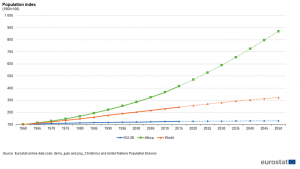Image 5: Statistics to Learn Essential Mathematical Skills

Statistics are the skeleton of Machine Learning. Everything you do in Machine Learning is statistics. Statistics are the mathematical DNA of every call of function you do when you are coding your ML model.

Since it is very important to understand statistics in order to build good Machine Learning models, here some of the concepts you should learn in order to have some basic coverage of the things that are going on there:

• Descriptive statistics: Central tendency, Mean, Median, Mode, Quantitative measures etc.
• Parameter estimation, Method of Moments, Maximum likelihood
• Confidence intervals, Hypothesis testing, Non-parametric tests
• Linear regression

Every concept and area above is a part of the day to day work of every data scientist.

### Two more books that are essential for learning more about Machine Learning and Data Science:

Introduction to Machine Learning with Python: A Guide for Data ScientistsBuy from Amazon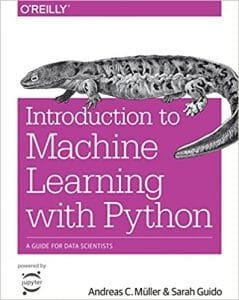Machine Learning For Absolute Beginners Buy from Amazon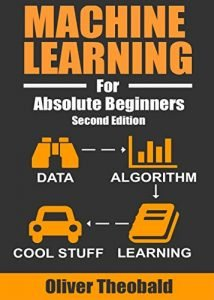## Conclusion

Data Science and Machine Learning were, are and will be part of every we do related to some sort of prediction or data analysis. The job positions in these fields skyrocketed in recent years as well as the salaries for these job positions. Many of these skills are required in order to get those positions.

As you can see the requirements are massive and complex, so if you are contemplating is you should apply for a job position in these areas, please read our “Data Science/Machine Learning job interview” post where you can what that experience looks like.

There are many courses online, as well as courses organized by academies that will help you understand these math skills in a short period of time, so you can cut the long process of learning them yourself.

Usually, those courses can be expensive, or might require a paying subscription. If you don’t want to pay any money, we suggest you subscribe to our website and receive notifications when we have a new post.

Please do follow us on our social media, since everything we do is free of charge forever, so we do need your support, by sharing our content with other people who want to learn new stuff for free.